Select Page

# CBSE Maths 12 Science MCQ Vector Algebra Solutions in English

CBSE Maths 12 Science MCQ Vector Algebra Solutions in English to enable students to get Solutions in a narrative video format for the specific question.

Expert Teacher provides CBSE Maths 12 Science MCQ Vector Algebra Solutions through Video Solutions in English language. This video solution will be useful for students to understand how to write an answer in exam in order to score more marks. This teacher uses a narrative style for a question from Vector Algebra not only to explain the proper method of answering question, but deriving right answer too.

Please find the question below and view the Solution in a narrative video format.

Question:

Solution Video in English:

You can select video Solutions from other languages also. Please check Solutions in ( Hindi )

## Similar Questions from CBSE, 12th Science, Maths, Vector Algebra

Question 1 : Find a unit vector parallel to the sum of vectors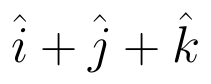and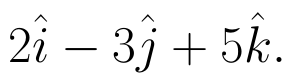(View Answer Video)

Question 2 : If the vertices A, B, C of a triangle ABC have position vectors (1, 2, 3), (-1, 0, 0), (0, 1, 2) respectively then find <ABC (<ABC is the angle between the vectors BA and BC). (View Answer Video)

Question 3 : Find the scalar quantities :
(i)     Work                     (ii)      Force

Question 4 :  If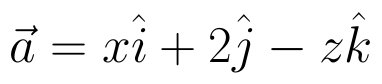and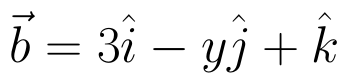are two equal vectors, then write the value of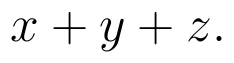(View Answer Video)

Question 5 : Find the vector quantities from the following:
(i) 10 kg          (ii) 2 m north-west          (iii)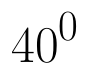(iv) 40 W         (v)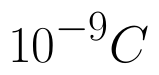(vi)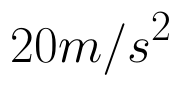(View Answer Video)

### Linear Programming

Question 1 : The objective function is maximum or minimum, which lies on the boundary of the feasible region. (View Answer Video)

### Application of Integrals

Question 1 : Find the area of the region bounded by the parabola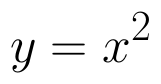and y = | x |. (View Answer Video)

Question 2 : Find the area of the region in the first quadrant enclosed by x-axis, line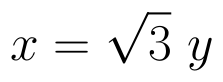and the circle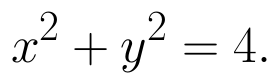(View Answer Video)

Question 3 : Using the method of integration find the area of the region bounded by lines:
2x + y = 4, 3x - 2y = 6 and x - 3y + 5 = 0.

Question 4 : Using integration, find the area of the triangle ABC, where A is (2,3), B is (4,7) and C is (6,2). (View Answer Video)

Question 5 : Using integration, find the area of the region bounded by the curves: y = |x + 1| + 1, x = -3, x = 3, y = 0. (View Answer Video)

### Integrals

Question 1 : Find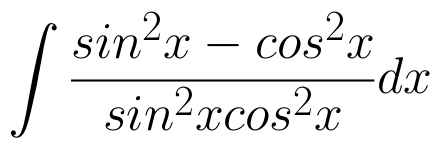. (View Answer Video)

Question 2 : Evaluate: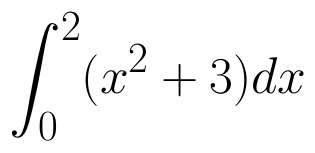as limit of sums.  (View Answer Video)

Question 3 : Find the integral of the function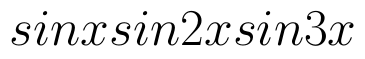. (View Answer Video)

Question 4 :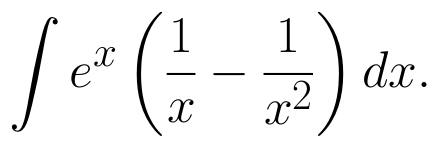(View Answer Video)

Question 5 : Evaluate: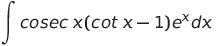(View Answer Video)